Next: Regression of lunar ascending Up: Lunar motion Previous: Summary of results

# Precession of lunar perigee

According to Equations (11.206) and (11.284), the mean ecliptic longitude of the lunar perigee evolves in time as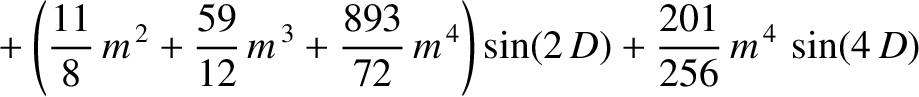(11.337)

where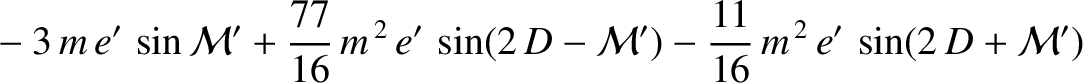(11.338)

Equation (11.337) implies that the perigee precesses (i.e., its longitude increases in time) at the mean rate of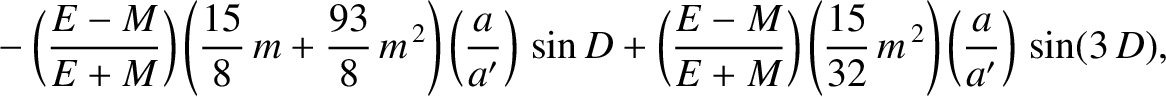degrees per year. (Of course, a year corresponds to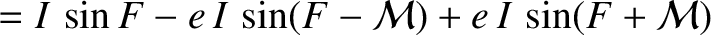.) Furthermore, it is clear that this precession is entirely due to the perturbing influence of the Sun, because it depends only on the parameter, which is a measure of this influence. Given that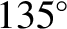, we find that the perigee advances by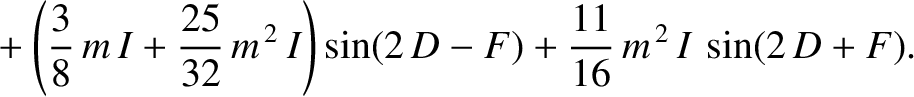degrees per year. Hence, we predict that the perigee completes a full circuit about the Earth every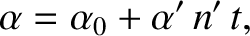years. In fact, the lunar perigee completes a full circuit every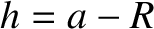years. Our prediction is somewhat inaccurate because our previous analysis neglected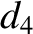, and smaller, contributions to the parameter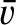[see Equation (11.284)], and these turn out to be significant. (See Section 11.17.)Next: Regression of lunar ascending Up: Lunar motion Previous: Summary of results
Richard Fitzpatrick 2016-03-31